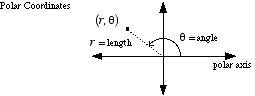# polar coordinates

Polar Coordinates

A way to describe the location of a point on a plane. A point is given coordinates (r, θ). r is the distance from the point to the origin. θ is the angle measured counterclockwise from the polar axis to the segment connecting the point to the origin.

Note: With polar coordinates a given point has many possible representations. θ has many possible values depending on which coterminal angle is chosen, and r can be positive or negative.